# US9793914B2 - Analog-to-digital converter, electronic device, and method of controlling analog-to-digital converter - Google Patents

Analog-to-digital converter, electronic device, and method of controlling analog-to-digital converter Download PDF

## Info

Publication number
US9793914B2
US9793914B2 US15/500,547 US201515500547A US9793914B2 US 9793914 B2 US9793914 B2 US 9793914B2 US 201515500547 A US201515500547 A US 201515500547A US 9793914 B2 US9793914 B2 US 9793914B2
Authority
US
United States
Prior art keywords
bit
change amount
delayed
current bit
greater
Prior art date
Legal status (The legal status is an assumption and is not a legal conclusion. Google has not performed a legal analysis and makes no representation as to the accuracy of the status listed.)
Active
Application number
US15/500,547
Other versions
US20170222655A1 (en
Inventor
Hiroshi Aoki
Ryuujirou Abe
Satoshi Fukuda
Current Assignee (The listed assignees may be inaccurate. Google has not performed a legal analysis and makes no representation or warranty as to the accuracy of the list.)
Sony Corp
Sony Semiconductor Solutions Corp
Original Assignee
Sony Corp
Priority date (The priority date is an assumption and is not a legal conclusion. Google has not performed a legal analysis and makes no representation as to the accuracy of the date listed.)
Filing date
Publication date
Priority to JP2014164568 priority Critical
Priority to JP2014-164568 priority
Application filed by Sony Corp filed Critical Sony Corp
Priority to PCT/JP2015/067297 priority patent/WO2016024439A1/en
Assigned to SONY SEMICONDUCTOR SOLUTIONS CORPORATION reassignment SONY SEMICONDUCTOR SOLUTIONS CORPORATION ASSIGNMENT OF ASSIGNORS INTEREST (SEE DOCUMENT FOR DETAILS). Assignors: ABE, Ryuujirou, AOKI, HIROSHI, FUKUDA, SATOSHI
Publication of US20170222655A1 publication Critical patent/US20170222655A1/en
Publication of US9793914B2 publication Critical patent/US9793914B2/en
Application granted granted Critical
Application status is Active legal-status Critical
Anticipated expiration legal-status Critical

• 230000001276 controlling effects Effects 0 claims description title 6
• 230000003111 delayed Effects 0 abstract claims description 143
• 230000001934 delay Effects 0 abstract claims description 9
• 230000000630 rising Effects 0 claims description 66
• 238000003708 edge detection Methods 0 claims description 48
• 230000000875 corresponding Effects 0 claims description 35
• 238000000034 methods Methods 0 claims description 6
• 238000006011 modification Methods 0 description 38
• 230000004048 modification Effects 0 description 38
• 239000000203 mixtures Substances 0 description 25
• 238000005070 sampling Methods 0 description 22
• 241001442055 Vipera berus Species 0 description 18
• 238000005516 engineering processes Methods 0 description 17
• 230000007274 generation of a signal involved in cell-cell signaling Effects 0 description 13
• 238000007792 addition Methods 0 description 7
• 230000000694 effects Effects 0 description 7
• 238000006243 chemical reaction Methods 0 description 5
• 239000002609 media Substances 0 description 2
• 239000007787 solids Substances 0 description 2
• 229920002574 CR-39 Polymers 0 description 1
• 239000008709 Curare Substances 0 description 1
• 230000002730 additional Effects 0 description 1
• 230000001721 combination Effects 0 description 1
• 238000004891 communication Methods 0 description 1
• 238000003384 imaging method Methods 0 description 1
• 230000001965 increased Effects 0 description 1
• 230000002829 reduced Effects 0 description 1
• 230000001603 reducing Effects 0 description 1
• 238000006722 reduction reaction Methods 0 description 1

## Images

•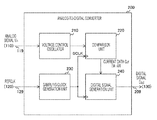•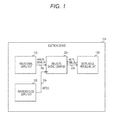•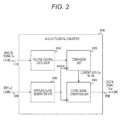•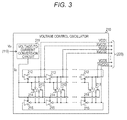•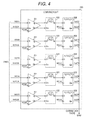•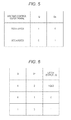•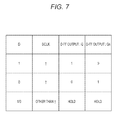•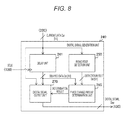•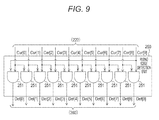•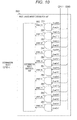••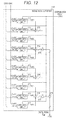•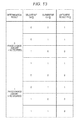•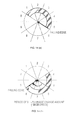•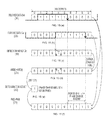•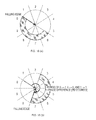•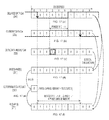•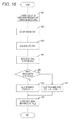••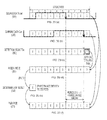•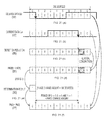•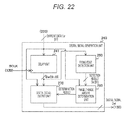•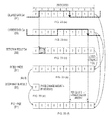•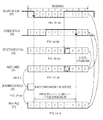•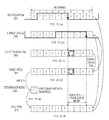•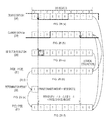## Classifications

• HELECTRICITY
• H03BASIC ELECTRONIC CIRCUITRY
• H03MCODING; DECODING; CODE CONVERSION IN GENERAL
• H03M1/00Analogue/digital conversion; Digital/analogue conversion
• H03M1/12Analogue/digital converters
• H03M1/124Sampling or signal conditioning arrangements specially adapted for A/D converters
• H03M1/1245Details of sampling arrangements or methods
• HELECTRICITY
• H03BASIC ELECTRONIC CIRCUITRY
• H03KPULSE TECHNIQUE
• H03K5/00Manipulating pulses not covered by one of the other main groups in this subclass
• H03K5/153Arrangements in which a pulse is delivered at the instant when a predetermined characteristic of an input signal is present or at a fixed time interval after this instant
• H03K5/1534Transition or edge detectors
• HELECTRICITY
• H03BASIC ELECTRONIC CIRCUITRY
• H03KPULSE TECHNIQUE
• H03K5/00Manipulating pulses not covered by one of the other main groups in this subclass
• H03K5/159Applications of delay lines not covered by the preceding subgroups
• HELECTRICITY
• H03BASIC ELECTRONIC CIRCUITRY
• H03MCODING; DECODING; CODE CONVERSION IN GENERAL
• H03M1/00Analogue/digital conversion; Digital/analogue conversion
• H03M1/12Analogue/digital converters
• H03M1/60Analogue/digital converters with intermediate conversion to frequency of pulses
• HELECTRICITY
• H03BASIC ELECTRONIC CIRCUITRY
• H03MCODING; DECODING; CODE CONVERSION IN GENERAL
• H03M1/00Analogue/digital conversion; Digital/analogue conversion
• H03M1/12Analogue/digital converters
• H03M1/64Analogue/digital converters with intermediate conversion to phase of sinusoidal or similar periodical signals

## Abstract

An analog signal is accurately converted into a digital signal. An oscillator generates an oscillation signal having a cycle that depends on a signal level of an input analog signal. A current bit generation unit generates, as a current bit, a bit indicating a value of the oscillation signal at each of a plurality of timings within the cycle. A delay unit delays each current bit over a predetermined period and supplies the delayed current bit as a delayed bit. A determination unit determines whether a change amount of a phase of the oscillation signal changed within the predetermined period is greater than a half cycle of the cycle. An output unit generates and outputs data indicating a period in which respective values of the current bit and the delayed bit form a specific combination when the change amount is not greater than the half cycle, and generates and outputs data indicating a period in which the respective values of the current bit and the delayed bit are the same or form the specific combination when the change amount is greater than the half cycle.

## Description

CROSS REFERENCE TO RELATED APPLICATIONS

This application is a U.S. National Phase of International Patent Application No. PCT/JP2015/067297 filed on Jun. 16, 2015, which claims priority benefit of Japanese Patent Application No. JP 2014-164568 filed in the Japan Patent Office on Aug. 12, 2014. Each of the above-referenced applications is hereby incorporated herein by reference in its entirety.

TECHNICAL FIELD

The present technology relates to an analog-to-digital converter, an electronic device, and a method of controlling the analog-to-digital converter. Specifically, the present technology relates to the analog-to-digital converter provided with an oscillator, the electronic device, and the method of controlling the analog-to-digital converter.

BACKGROUND ART

Conventionally, when an analog signal is processed in a digital circuit, an analog-to-digital (AD) converter for converting the analog signal into a digital signal is provided at a preceding stage of the digital circuit. For example, an AD converter provided with an oscillator that generates an oscillation signal having a frequency that depends on a signal level of an analog signal, n (n is an integer of two or more) flip-flops, and a logic circuit has been proposed (for example, refer to Non-Patent Document 1).

In the AD converter, the n flip-flops hold values of the oscillation signal at respective timings obtained by dividing an oscillation cycle of the oscillation signal into n, and supply data indicating these values to the logic circuit. Then, the logic circuit delays the data over a period of one cycle of a sampling clock, compares the data before the delay and the data after the delay on a bit basis to count the number of bits changed from “0” to “1”, and outputs a digital signal of the count value. If a change amount of a phase of the oscillation signal within the sampling period is equal to or less than a half cycle of the oscillation cycle, the count value depends on the change amount of the phase. Therefore, the digital signal has an accurate value that depends on the signal level of the analog signal.

CITATION LIST Non-Patent Document

Non-Patent Document 1: Matthew Park, et al., A 78 dB SNDR 87 mW 20 MHz Bandwidth Continuous-Time ΔΣ ADC with VCO-Based integrator and quantizer implemented in 0.13 um CMOS, IEEE Vol. 44.

SUMMARY OF THE INVENTION Problems to be Solved by the Invention

However, the above-mentioned conventional technology might not be able to convert the analog signal into the digital signal having the accurate value. This is because the accurate value that depends on the signal level of the analog signal cannot be obtained if the change amount of the phase exceeds the half cycle of the oscillation cycle. Therefore, it is sometimes the case that the digital signal having the accurate value is not output when the phase change amount might exceed the half cycle of the oscillation cycle, the result of which leads to a problem of a reduction in the signal-to-noise (S/N) ratio of the AD converter.

The present technology has been created in view of the above circumstances, and an object thereof is to accurately convert an analog signal into a digital signal.

Solutions to Problems

The present technology has been made in order to solve the above-mentioned problem. A first aspect of the present technology is an analog-to-digital converter and a method of controlling the same, and the analog-to-digital converter includes: an oscillator that generates an oscillation signal having a cycle that depends on a signal level of an input analog signal; a current bit generation unit that generates, as a current bit, a bit indicating a value of the oscillation signal at each of a plurality of timings within the cycle; a delay unit that delays each current bit over a predetermined period and supplies the delayed current bit as a delayed bit; a determination unit that determines whether a change amount of a phase of the oscillation signal changed within the predetermined period is greater than a half cycle of the cycle; and an output unit that generates and outputs data indicating a period in which respective values of the current bit and the delayed bit form a specific combination when the change amount is not greater than the half cycle, and generates and outputs data indicating a period in which the respective values of the current bit and the delayed bit are the same or form the specific combination when the change amount is greater than the half cycle. This provides a function of outputting the data indicating the period in which the respective values of the current bit and the delayed bit are the same or form the specific combination when the change amount of the phase of the oscillation signal changed within the predetermined period is greater than the half cycle.

In addition, the first aspect may further include an edge detection unit that detects presence or absence of one of a rising edge and a falling edge of the oscillation signal at each of the plurality of timings on the basis of either the current bit or the delayed bit, and the determination unit may detect whether the change amount is great as compared with the half cycle in accordance with whether the respective values of the current bit and the delayed bit corresponding to the timing at which the presence of one of the edges is detected are the same. This provides a function of detecting whether the phase change amount is great as compared with the half cycle in accordance with whether the respective values of the current bit and the delayed bit corresponding to the timing at which the presence of the edge is detected are the same.

In addition, according to the first aspect, the edge detection unit may detect the presence or absence of one of the edges on the basis of the delayed bit. This provides a function of detecting the presence or absence of one of the edges on the basis of the delayed bit.

In addition, according to the first aspect, the edge detection unit may detect the presence or absence of the rising edge on the basis of the delayed bit, and the determination unit may determine that the change amount is not greater than the half cycle when the current bit corresponding to the timing at which the presence of the rising edge is detected is at a low level, and may determine that the change amount is greater than the half cycle when the current bit is at a high level. This provides a function of determining that the change amount is not greater than the half cycle when the current bit corresponding to the timing at which the presence of the rising edge is detected is at the low level.

In addition, according to the first aspect, the edge detection unit may detect the presence or absence of the falling edge on the basis of the delayed bit, and the determination unit may determine that the change amount is not greater than the half cycle when the current bit corresponding to the timing at which the presence of the falling edge is detected is at a high level, and may determine that the change amount is greater than the half cycle when the current bit is at a low level. This provides a function of determining that the change amount is not greater than the half cycle when the current bit corresponding to the timing at which the presence of the falling edge is detected is at the high level.

In addition, according to the first aspect, the edge detection unit may detect the presence or absence of one of the edges on the basis of the current bit. This provides a function of detecting the presence or absence of one of the edges on the basis of the current bit.

In addition, according to the first aspect, the edge detection unit may detect the presence or absence of the rising edge on the basis of the current bit, and the determination unit may determine that the change amount is not greater than the half cycle when the delayed bit corresponding to the timing at which the presence of the rising edge is detected is at a high level, and may determine that the change amount is greater than the half cycle when the delayed bit is at a low level. This provides a function of determining that the change amount is not greater than the half cycle when the delayed bit corresponding to the timing at which the presence of the rising edge is detected is at the high level.

In addition, according to the first aspect, the edge detection unit may detect the presence or absence of the falling edge on the basis of the current bit, and the determination unit may determine that the change amount is not greater than the half cycle when the delayed bit corresponding to the timing at which the presence of the falling edge is detected is at a low level, and may determine that the change amount is greater than the half cycle when the delayed bit is at a high level. This provides a function of determining that the change amount is not greater than the half cycle when the delayed bit corresponding to the timing at which the presence of the rising edge is detected is at the low level.

In addition, according to the first aspect, the output unit may count the number of the timings at which the specific combination is formed and generate data of a count value as the data indicating the period when the change amount is not greater than the half cycle, and may count the number of the timings at which the respective values of the current bit and the delayed bit are the same or form the specific combination and generate data of a count value as the data indicating the period when the change amount is greater than the half cycle. This provides a function of counting the number of timings at which the respective values of the current bit and the delayed bit are the same or form the specific combination when the change amount is greater than the half cycle.

Moreover, a second aspect of the present technology is an electronic device including: an oscillator that generates an oscillation signal having a cycle that depends on a signal level of an input analog signal; a current bit generation unit that generates, as a current bit, a bit indicating a value of the oscillation signal at each of a plurality of timings within the cycle; a delay unit that delays each current bit over a predetermined period and supplies the delayed current bit as a delayed bit; a determination unit that determines whether a change amount of a phase of the oscillation signal changed within the predetermined period is greater than a half cycle of the cycle; an output unit that generates and outputs data indicating a period in which respective values of the current bit and the delayed bit form a specific combination when the change amount is not greater than the half cycle, and generates and outputs data indicating a period in which the respective values of the current bit and the delayed bit are the same or form the specific combination when the change amount is greater than the half cycle; and a processing unit that processes the data. This provides a function of outputting the data indicating the period in which the respective values of the current bit and the delayed bit are the same or form the specific combination when the change amount of the phase of the oscillation signal changed within the predetermined period is greater than the half cycle.

Effects of the Invention

According to the present technology, an excellent effect of being capable of accurately converting an analog signal into a digital signal can be achieved. Note that the effects described herein are not necessarily limited, and any of effects described in the present disclosure may be obtained.

BRIEF DESCRIPTION OF DRAWINGS

FIG. 1 is a block diagram illustrating a configuration example of an electronic device according to an embodiment.

FIG. 2 is a block diagram illustrating a configuration example of an analog-to-digital converter according to the embodiment.

FIG. 3 is a circuit diagram illustrating a configuration example of a voltage control oscillator according to the embodiment.

FIG. 4 is a circuit diagram illustrating a configuration example of a comparison unit according to the embodiment.

FIG. 5 is a table illustrating an example of operation of a comparator according to the embodiment.

FIG. 6 is a table illustrating an example of operation of a latch circuit according to the embodiment.

FIG. 7 is a truth table illustrating an example of operation of a flip-flop according to the embodiment.

FIG. 8 is a block diagram illustrating a configuration example of a digital signal output unit according to the embodiment.

FIG. 9 is a circuit diagram illustrating a configuration example of a rising edge detection unit according to the embodiment.

FIG. 10 is a circuit diagram illustrating a configuration example of a phase change amount determination unit according to the embodiment.

FIG. 11 is a table illustrating an example of operation of a determination result generation unit according to the embodiment.

FIG. 12 is a circuit diagram illustrating a configuration example of a digital signal generation unit according to the embodiment.

FIG. 13 is a table illustrating an example of operation of an arithmetic circuit according to the embodiment.

FIGS. 14(a) and 14(b) are diagrams illustrating examples of respective edge positions indicated by delayed data and current data when a phase change amount is equal to or less than 180 degrees according to the embodiment.

FIGS. 15(a) to 15(f) are diagrams for explaining an arithmetic method that is applied when the phase change amount is equal to or less than 180 degrees according to the embodiment.

FIGS. 16(a) and 16(b) are diagrams illustrating examples of the respective edge positions indicated by the delayed data and the current data when the phase change amount exceeds 180 degrees according to the embodiment.

FIGS. 17(a) to 17(f) are diagrams for explaining an arithmetic method that is applied when the phase change amount exceeds 180 degrees according to the embodiment.

FIG. 18 is a flowchart illustrating an example of operation of the analog-to-digital converter according to the embodiment.

FIG. 19 is a circuit diagram illustrating a configuration example of a falling edge detection unit according to a first modification of the embodiment.

FIGS. 20(a) to 20(f) are diagrams for explaining an arithmetic method that is applied when the phase change amount is equal to or less than 180 degrees according to the first modification of the embodiment.

FIGS. 21(a) to 21(f) are diagrams for explaining an arithmetic method that is applied when the phase change amount exceeds 180 degrees according to the first modification of the embodiment.

FIG. 22 is a block diagram illustrating a configuration example of the digital signal generation unit according to a second modification of the embodiment.

FIGS. 23(a) to 23(f) are diagrams for explaining an arithmetic method that is applied when the phase change amount is equal to or less than 180 degrees according to the second modification of the embodiment.

FIGS. 24(a) to 24(f) are diagrams for explaining an arithmetic method that is applied when the phase change amount exceeds 180 degrees according to the second modification of the embodiment.

FIGS. 25(a) to 25(f) are diagrams for explaining an arithmetic method that is applied when the phase change amount is equal to or less than 180 degrees according to a third modification of the embodiment.

FIGS. 26(a) to 26(f) are diagrams for explaining an arithmetic method that is applied when the phase change amount exceeds 180 degrees according to the third modification of the embodiment.

MODE FOR CARRYING OUT THE INVENTION

Hereinafter, a mode for carrying out the present technology (hereinafter referred to as an embodiment) will be described. The description will proceed in the following order.

1. First Embodiment (example of performing AD conversion after determining whether a phase change amount is equal to or less than a half cycle)

2. First Modification

3. Second Modification

4. Third Modification

1. First Embodiment

[Configuration Example of Electronic Device]

FIG. 1 is a block diagram illustrating a configuration example of an electronic device 100 according to an embodiment of the present technology. The electronic device 100 is a device for converting an analog signal into a digital signal and processing the digital signal, and includes an analog signal supply unit 110, a reference clock supply unit 120, an analog-to-digital converter 200, and a digital signal processing unit 130. As the electronic device 100, for example, an imaging device, a wireless communication device, a measuring device, and an information processing device or the like are assumed.

Note that although the analog-to-digital converter 200 is herein configured to be provided in a device such as the electronic device 100, the analog-to-digital converter 200 may be provided in a module or an instrument such as an image sensor or a measuring instrument.

The analog signal supply unit 110 generates an analog voltage signal as an analog signal Vin, and supplies the analog signal Vin to the analog-to-digital converter 200 via a signal line 119. For example, an audio signal or an image signal is generated as the analog signal Vin. Note that the analog signal supply unit may generate an analog current signal. The analog signal supply unit 110 may also receive an analog signal from the outside and supply the analog signal to the analog-to-digital converter 200.

The reference clock supply unit 120 generates a reference clock signal REFCLK having a predetermined frequency as a clock signal to be referred to, and supplies the reference clock signal REFCLK to the analog-to-digital converter 200 via a signal line 129.

The analog-to-digital converter 200 converts the analog signal Vin into a digital signal Dout in synchronization with the reference clock signal REFCLK. The analog-to-digital converter 200 supplies the converted digital signal Dout to the digital signal processing unit 130 via a signal line 209.

The digital signal processing unit 130 performs a predetermined process such as an image process and an audio process on the digital signal Dout. Note that the digital signal processing unit 130 is an example of a processing unit described in the claims.

[Configuration Example of Analog-to-Digital Converter]

FIG. 2 is a block diagram illustrating a configuration example of the analog-to-digital converter 200 according to the embodiment. The analog-to-digital converter 200 includes a voltage control oscillator 210, a comparison unit 220, a sampling clock generation unit 230, and a digital signal generation unit 240.

The voltage control oscillator 210 generates a plurality of voltage control oscillation signals having a frequency that depends on a signal level of the analog signal Vin. For example, ten clock signals, i.e. VCO1 to VCO5 and XVCO1 to XVCO5, are generated as the voltage control oscillation signals. These voltage control oscillation signals are signals having the same frequency and phases different from one another. The voltage control oscillator 210 supplies these voltage control oscillation signals to the comparison unit 220. Note that the voltage control oscillator 210 is an example of an oscillator described in the claims. In addition, the voltage control oscillation signal is an example of an oscillation signal described in the claims.

Note that a current control oscillator that generates an oscillation signal having a frequency that depends on a level of the current may be provided in place of the voltage control oscillator. In this case, the current signal is input to the analog-to-digital converter 200 as the analog signal.

The sampling clock generation unit 230 generates, as a sampling clock signal SCLK, a clock signal having a higher frequency than the reference clock signal REFCLK. For example, the sampling clock signal SCLK is generated by multiplying the reference clock signal REFCLK.

The comparison unit 220 compares, in synchronization with the sampling clock signal SCLK, two voltage control oscillation signals (e.g. VCO1 and XVCO1) having phases different from each other, and generates current data Cur including a plurality of (e.g. ten) bits from a comparison result. Each of the bits in the current data Cur indicates a value of the voltage control oscillation signal at each of timings obtained by equally dividing a cycle of the voltage control oscillation signal (for example, into ten). These bits are hereinafter referred to as “current bits”. The comparison unit 220 supplies the current data Cur to the digital signal generation unit 240. Note that the comparison unit 220 is an example of a current bit generation unit described in the claims.

The digital signal generation unit 240 generates the digital signal Dout from the current data Cur in synchronization with the sampling clock signal SCLK. Details of a method of generating the digital signal Dout will be described later.

[Configuration Example of Voltage Control Oscillator]

FIG. 3 is a circuit diagram illustrating a configuration example of the voltage control oscillator 210 according to the embodiment. The voltage control oscillator 210 includes a voltage-to-current conversion circuit 211, an odd number of inverters 212, an odd number of inverters 213, an odd number of inverters 214, and an odd number of inverters 215. The number of respective inverters is, for example, five.

The voltage-to-current conversion circuit 211 converts the voltage of the analog signal Vin into the current. The voltage-to-current conversion circuit 211 supplies the converted current signal Iin to each of the inverters 212, 213, 214, and 215.

The five inverters 212 are connected in a ring shape, and so are the five inverters 215. The inverters 213 and 214 are connected to the inverters 212 different from one another and to the inverters 215 different from one another. For example, an input terminal of the inverter 213 is connected to an output terminal of the inverter 212, and an output terminal of the inverter 213 is connected to an output terminal of the inverter 215. Moreover, an input terminal of the inverter 214 is connected to the output terminal of the inverter 215, and an output terminal of the inverter 214 is connected to the output terminal of the inverter 212.

Owing to this connection, the inverter ring including the inverters 212 and the inverter ring including the inverters 215 are connected by latches including the inverters 213 and 214. Accordingly, differential symmetry of output waveforms of the respective inverter rings is increased, whereby the duty ratio of a waveform of an output signal is easily maintained at 50%.

In this configuration, the inverter 212 generates the voltage control oscillation signal having a frequency that depends on the level of the current signal Iin. Meanwhile, the inverter 215 generates the clock signal having the same frequency as the voltage control oscillation signal generated by the inverter 212 and having a phase different from that of the voltage control oscillation signal generated by the inverter 212 by a half cycle (in other words, having an inverted phase).

The five inverters 212 supply the voltage control oscillation signals VCO1 to VCO5 to the comparison unit 220, and the five inverters 215 supply the voltage control oscillation signals XVCO1 to XVCO5 to the comparison unit 220.

[Configuration Example of Comparison Unit]

FIG. 4 is a circuit diagram illustrating a configuration example of the comparison unit 220 according to the embodiment. The comparison unit 220 includes an odd number of comparators 221, an odd number of latch circuits 222, and an odd number of flip-flops 223. For example, the number of comparators 221, the number of latch circuits 222, and the number of flip-flops 223 are all five.

The comparator 221 compares the two voltage control oscillation signals having the phases different from each other. The comparator 221 also includes a clock terminal, input terminals D and Dn, and output terminals Q and Qn. The sampling clock signal SCLK is input to the clock terminal. In addition, the voltage control oscillation signal VCOi is input to the input terminal D of the i-th (i is an integer of one to five) comparator 221, and the voltage control oscillation signal XVCOi is input to the input terminal Dn of the i-th comparator 221.

The comparator 221 compares respective levels of the voltage control oscillation signal VCOi and the voltage control oscillation signal XVCOi in synchronization with the sampling clock signal SCLK, and outputs the comparison result as a set signal from the output terminal Q. For example, a high level set signal is output when the voltage control oscillation signal VCOi is higher than the voltage control oscillation signal XVCOi, and a low level set signal is output in the other case. In addition, a signal obtained by inverting the set signal is output as a reset signal from the output terminal Qn.

The latch circuit 222 outputs the comparison result on the basis of the set signal and the reset signal from the comparator 221. In addition, the latch circuits 222 are connected to the comparators 221 different from one another, and each of the latch circuits 222 includes a set terminal D, a reset terminal Dn, and an output terminal Q. The set signal from the corresponding comparator 221 is input to the set terminal D, and the reset signal is input to the reset terminal Dn. The latch circuit 222 outputs a high level signal from the output terminal Q when the set signal is at a high level and the reset signal is at a low level, and outputs a low level signal from the output terminal Q when the set signal is at a low level and the reset signal is at a high level.

The flip-flops 223 hold the current bits. In addition, the flip-flops 223 are connected to the latch circuits 222 different from one another, and each of the flip-flops 223 includes a clock terminal, an input terminal D, and output terminals Q and Qn. The sampling clock signal SCLK is input to the clock terminal, and the input terminal D of the flip-flop 223 is connected to the output terminal Q of the corresponding latch circuit 222. The i-th flip-flop 223 holds the signal from the corresponding latch circuit 222 as a current bit Cur [i−1], and outputs the current bit Cur [i−1] from the output terminal Q in synchronization with the sampling clock signal SCLK. The flip-flop 223 also outputs a signal obtained by inverting the current bit Cur[i−1] as a current bit Cur[i+4] from the output terminal Qn. As a result, the current data Cur including the current bits Cur  to Cur  are output from the five flip-flops 223 to the digital signal generation unit 240.

FIG. 5 is a table illustrating an example of the operation of the comparator 221 according to the embodiment. The comparator 221 outputs the set signal “1” from the output terminal Q when the voltage control oscillation signal VCOi is higher than the voltage control oscillation signal XVCOi, and outputs the set signal “0” from the output terminal Q in the other case. The comparator 221 also outputs the reset signal obtained by inverting the set signal from the output terminal Qn.

FIG. 6 is a table illustrating an example of the operation of the latch circuit 222 according to the embodiment. The latch circuit 222 outputs the signal “0” from the output terminal Q when the set signal input to the input terminal D is “0” and the reset signal input to the input terminal Dn is “1”. The latch circuit 222 also outputs the signal “1” from the output terminal Q when the set signal is “1” and the reset signal is “0”. In addition, when both the set signal and the reset signal are “0”, the latch circuit 222 shifts to a hold state in which the value of the output terminal Q is held.

FIG. 7 is a truth table illustrating an example of the operation of the flip-flop 223 according to the embodiment. The flip-flop 223 outputs the signal from the input terminal D as the current bit Cur [i−1] from the output terminal Q at the time of a rising edge of the sampling clock signal SCLK. The flip-flop 223 also holds the state of the output terminal Q in a period other than the rising edge of the sampling clock signal SCLK. The flip-flop 223 also outputs the signal obtained by inverting the current bit Cur [i−1] from the output terminal Qn.

[Configuration Example of Digital Signal Generation Unit]

FIG. 8 is a block diagram illustrating a configuration example of the digital signal generation unit 240 according to the embodiment. The digital signal generation unit 240 includes a delay unit 241, a rising edge detection unit 250, a phase change amount determination unit 260, and a digital signal output unit 270.

The delay unit 241 delays the current data Cur over a cycle of the sampling clock signal SCLK. The delay unit 241 supplies the delayed current data Cur as delayed data Del to the phase change amount determination unit 260 and the digital signal output unit 270. Each bit in the delayed data Del is hereinafter referred to as a “delayed bit”.

The rising edge detection unit 250 detects, on the basis of the current data Cur, the presence or absence of a rising edge of the voltage control oscillation signal VCO at each of the plurality of (e.g. ten) timings within the cycle of the voltage control oscillation signal VCO. The rising edge detection unit 250 supplies a detection result Det to the phase change amount determination unit 260. The detection result Det includes a plurality of detection bits [j]. As used herein, j is, for example, an integer of zero to nine. For example, a value “1” is set to the detection bit for the timing at which the presence of the rising edge is detected, and values “0” are set to the other detection bits. Note that the rising edge detection unit 250 is an example of an edge detection unit described in the claims.

The phase change amount determination unit 260 determines whether a change amount of the phase of the voltage control oscillation signal VCO (hereinafter referred to as a “phase change amount”) in the cycle of the sampling clock signal SCLK is greater than a half cycle (180 degrees in angle notation) of the cycle. The phase change amount determination unit 260 determines whether the phase change amount is great as compared with the half cycle in accordance with whether values of the current bit and the delayed bit corresponding to the timing at which the presence of the rising edge is detected are the same. For example, when the values of the current bit and the delayed bit are the same, it is determined that the phase change amount is equal to or less than the half cycle. When the values are different, it is determined that the phase change amount is greater than the half cycle. The phase change amount determination unit 260 supplies a determination result to the digital signal output unit 270.

Note that the phase change amount determination unit 260 is an example of a determination unit described in the claims.

The digital signal output unit 270 generates and outputs the digital signal Dout on the basis of the current data Cur, the delayed data Del, and the determination result. When the phase change amount is equal to or less than the half cycle, the digital signal output unit 270 generates the digital signal Dout indicating a period in which the respective values of the delayed bit and the current bit form a specific combination. For example, the digital signal Dout indicating the period in which the delayed bit is “0” and the current bit is “1” is generated.

On the other hand, when the phase change amount is greater than the half cycle, the digital signal output unit 270 generates the digital signal Dout indicating a period in which the respective values of the delayed bit and the current bit are the same or form the aforementioned specific combination. For example, the digital signal Dout indicating the period in which both the delayed bit and the current bit are “0” or “1”, or the delayed bit is “0” and the current bit is “1” is generated. The digital signal output unit 270 outputs the generated digital signal Dout to the digital signal processing unit 130. Note that the digital signal output unit 270 is an example of an output unit described in the claims.

[Configuration Example of Rising Edge Detection Unit]

FIG. 9 is a circuit diagram illustrating a configuration example of the rising edge detection unit 250 according to the embodiment. The rising edge detection unit 250 includes a plurality of (e.g. ten) AND (logical conjunction) gates 251.

The AND gate 251 outputs the logical conjunction of two logical values. The zeroth AND gate 251 outputs the logical conjunction of a value obtained by inverting the current bit Cur and a value of the current bit Cur  as the detection bit Det . The j-th AND gate 251 including the first and subsequent AND gates 251 outputs the logical conjunction of a value obtained by inverting the current bit Cur[j−1] and a value of the current bit Cur[j] as the detection bit Det[j].

[Configuration Example of Phase Change Amount Determination Unit]

FIG. 10 is a circuit diagram illustrating a configuration example of the phase change amount determination unit 260 according to the embodiment. The phase change amount determination unit 260 includes a plurality of (e.g. ten) AND gates 261 and a determination result generation unit 262.

The AND gate 261 outputs the logical conjunction of two logical values. The j-th AND gate 261 supplies the logical conjunction of the delayed bit Del[j] and the detection bit Det[j] as AND[j] to the determination result generation unit 262.

The determination result generation unit 262 generates the determination result on the basis of AND to AND. The fact that all of AND to AND are “0” indicates that the values of the current bit and the delayed bit corresponding to the timing at which the presence of the rising edge is detected are different. In this case, the determination result generation unit 262 determines that the phase change amount is greater than the half cycle, and generates, for example, the determination result indicating a value “0”.

On the other hand, the fact that any of AND to AND is “1” indicates that the values of the current bit and the delayed bit corresponding to the timing at which the presence of the rising edge is detected are the same. In this case, the determination result generation unit 262 determines that the phase change amount is equal to or less than the half cycle, and generates, for example, the determination result indicating a value “1”.

FIG. 11 is a table illustrating an example of the operation of the determination result generation unit 262 according to the embodiment. When all of AND to AND are “0”, the determination result generation unit 262 determines that the phase change amount is greater than the half cycle, and generates, for example, the determination result indicating the value “0”. On the other hand, when any of AND to AND is “1”, the determination result generation unit 262 determines that the phase change amount is equal to or less than the half cycle, and generates, for example, the determination result indicating the value “1”.

Note that the determination result generation unit 262 may generate the determination result indicating the value “1” when it is determined that the phase change amount is greater than the half cycle, and may generate the determination result indicating the value “0” in the other case.

[Configuration Example of Digital Signal Output Unit]

FIG. 12 is a circuit diagram illustrating a configuration example of the digital signal output unit 270 according to the embodiment. The digital signal output unit 270 includes a plurality of (e.g. ten) arithmetic circuits 271 and adders 272 to 278.

The arithmetic circuit 271 judges whether the values of the current bit Cur[j] and the delayed bit Del[j] are the same or form the specific combination when the phase change amount is greater than the half cycle, and generates a bit indicating a judgment result as an arithmetic result Ph[j]. The specific combinations is, for example, a combination of the delayed bit Del[j] indicating “0” and the current bit Cur[j] indicating “1”. In addition, for example, a value “1” is set to the arithmetic result Ph[j] when the values of the current bit Cur[j] and the delayed bit Del[j] are the same or form the specific combination, and a value “0” is set to the arithmetic result Ph[j] in the other case.

On the other hand, the arithmetic circuit 271 judges whether the values of the current bit [j] and the delayed bit Del[j] form the specific combination when the phase change amount is equal to or less than the half cycle, and generates a bit indicating a judgment result as an arithmetic result Ph[h]. For example, a value “1” is set to the arithmetic result Ph[j] when the values of the current bit Cur [j] and the delayed bit Del [j] form the specific combination, and a value “0” is set to the arithmetic result Ph[j] in the other case.

FIG. 13 is a table illustrating an example of the operation of the arithmetic circuit 271 according to the embodiment. First, a case where the determination result has the value “0”, that is, the phase change amount is greater than 180 degrees, is considered. In this case, the arithmetic circuit 271 generates the arithmetic result Ph[j] indicating “1” if either a condition that the delayed bit Del[j] is “0” and the current bit [j] is “1” or a condition that the values of the delayed bit Del[j] and the current bit [j] are the same is satisfied. On the other hand, the arithmetic circuit 271 generates the arithmetic result Ph[j] indicating “0” if these conditions are not satisfied.

To the contrary, in a case where the phase change amount is equal to or less than 180 degrees, the arithmetic result Ph[j] is generated using a different logical operation. In this case, the arithmetic circuit 271 generates the arithmetic result Ph[j] indicating “1” when the delayed bit Del[j] is “0” and the current bit [j] is “1”, and generates the arithmetic result Ph[j] indicating “0” in the other case.

FIGS. 14(a) and 14(b) are diagrams illustrating examples of respective edge positions indicated by the delayed data and the current data when the phase change amount is equal to or less than 180 degrees according to the embodiment. In FIGS. 14(a) and 14(b), an angle, to a specific straight light, of each of ten straight lines extending radially from the center of the circle indicates the phase at each of the timings obtained by dividing the cycle of the voltage control oscillation signal into ten. Since 360 degrees are divided into ten, the respective timings are different from each other by 36 degrees. In addition, a section with diagonal lines indicates a period in which the value of the voltage control oscillation signal is “1”.

A diagram of an example of the timing of a falling edge of the voltage control oscillation signal VCO indicated by the delayed data is illustrated in FIG. 14(a). In this example, the fifth timing is the timing of the falling edge. In addition, a diagram of an example of the timing of the falling edge of the voltage control oscillation signal VCO indicated by the current data is illustrated in FIG. 14(b). In this example, the eighth timing is the falling edge. From these results, the phase change amount within the period of the sampling clock signal SCLK is 108 (=3×36) degrees. The phase change amount is equal to a period in which the value of the voltage control oscillation signal VCO is changed from “0” to “1”.

FIGS. 15(a) to 15(f) are diagrams for explaining an arithmetic method that is applied when the phase change amount is equal to or less than 180 degrees according to the embodiment. A diagram of an example of the delayed data Del generated by the delay unit 241 is illustrated in FIG. 15(a). In FIG. 15(a), a thick solid line indicates a waveform of the voltage control oscillation signal VCO. In addition, the delayed data Del include the ten delayed bits, and each bit indicates the value of the voltage control oscillation signal VCO at each of the timings obtained by dividing the cycle of the voltage control oscillation signal VCO into ten.

A diagram of an example of the current data Cur generated by the comparison unit 220 is illustrated in FIG. 15(b). In FIG. 15(b), a thick solid line indicates a waveform of the voltage control oscillation signal VCO. In addition, the current data Cur include the ten current bits, and each bit indicates the value of the voltage control oscillation signal VCO at each of the timings obtained by dividing the cycle of the voltage control oscillation signal VCO into ten.

A diagram of an example of the detection result Det of the rising edge detected from the current data by the rising edge detection unit 250 is illustrated in FIG. 15(c). The detection result Det includes the ten detection bits, and each bit indicates the presence or absence of the rising edge at each of the timings obtained by dividing the cycle of the voltage control oscillation signal VCO into ten. In this example, the presence of the rising edge is detected at the fourth detection bit.

A diagram of an example of AND to AND generated by the AND gates 261 is illustrated in FIG. 15(d). The logical conjunction of the delayed bit and the detection result bit is generated as each of AND to AND. Since the presence of the rising edge is detected at the fourth bit, and the fourth delayed bit is also “1”, AND which is the logical conjunction of them has the value “1”. The fact that AND is “1” indicates that the current bit and the delayed bit corresponding to the fourth timing at which the presence of the rising edge is detected have the same value.

A diagram of an example of the determination result generated by the determination result generation unit 262 is illustrated in FIG. 15(e). Since AND is “1”, the determination result “1” indicating that the phase change amount is equal to or less than 180 degrees is generated.

A diagram of an example of the arithmetic results Ph[j] provided by the arithmetic circuits 271 is illustrated in FIG. 15(f). When the phase change amount is equal to or less than 180 degrees, the arithmetic circuit 271 generates the arithmetic result “j” indicating the value “1” at the timing at which the delayed bit is “0” and the current bit is “1”. In this example, since the delayed bits are “0” and the current bits are “1” at the sixth to eighth timings, the arithmetic results Ph “6” to Ph “8” indicating the values “1” are generated. The period “3” including these timings is calculated as the phase change amount.

FIGS. 16(a) and 16(b) are diagrams illustrating examples of the respective edge positions indicated by the delayed data and the current data when the phase change amount is greater than 180 degrees according to the embodiment. A diagram of an example of the timing of the falling edge of the voltage control oscillation signal VCO indicated by the delayed data is illustrated in FIG. 16(a). In this example, the zeroth timing is the timing of the falling edge. In addition, a diagram of an example of the timing of the falling edge of the voltage control oscillation signal VCO indicated by the current data is illustrated in FIG. 16(b). In this example, the seventh timing is the falling edge. From these results, the phase change amount within the period of the sampling clock signal SCLK is 252 (=7×36) degrees. The phase change amount is equal to the sum of a period in which the value of the voltage control oscillation signal VCO is changed from “0” to “1” and a period in which the value is not changed.

FIGS. 17(a) to 17(f) are diagrams for explaining an arithmetic method that is applied when the phase change amount is greater than 180 degrees according to the embodiment. A diagram of an example of the delayed data Del generated by the delay unit 241 is illustrated in FIG. 17(a). A diagram of an example of the current data Cur generated by the comparison unit 220 is illustrated in FIG. 17(b).

A diagram of an example of the detection result Det of the rising edge detected from the current data by the rising edge detection unit 250 is illustrated in FIG. 17(c). In this example, the presence of the rising edge is detected at the third detection bit.

A diagram of an example of AND to AND generated by the AND gates 261 is illustrated in FIG. 17(d). Since the presence of the rising edge is detected at the third bit, and the third delayed bit is “0”, AND which is the logical conjunction of them has the value “0”. The fact that AND is “0” indicates that the current bit and the delayed bit corresponding to the third timing at which the presence of the rising edge is detected have the different values.

A diagram of an example of the determination result generated by the determination result generation unit 262 is illustrated in FIG. 17(e). Since all the values of AND[j] are “0”, the determination result “0” indicating that the phase change amount is greater than 180 degrees is generated.

A diagram of an example of the arithmetic results Ph[j] provided by the arithmetic circuits 271 is illustrated in FIG. 17(f). When the phase change amount is greater than 180 degree, the arithmetic circuit 271 generates the arithmetic result Ph “j” indicating the value “1” at the timing at which the delayed bit is “0” and the current bit is “1”, and the timing at which the values of the delayed bit and the current bit are the same. In this example, the arithmetic results Ph “1” to Ph “7” indicating the values “1” are generated at the first to seventh timings. The period “7” including these timings is calculated as the phase change amount.

If the period in which the delayed bit is “0” and the current bit is “1” is obtained without the determination as to whether the phase change amount is greater than 180 degrees, the phase change amount “3” is calculated from FIGS. 15(a) and 15(b). This phase change amount is not the accurate value (“7”). As a result, the S/N ratio of the analog-to-digital converter might be reduced.

To the contrary, the analog-to-digital converter 200 according to the embodiment switches the arithmetic method in accordance with whether the phase change amount within the sampling period is greater than 180 in the clock signal having the cycle that depends on the signal level of the analog signal. Therefore, the analog-to-digital converter 200 can calculate the accurate phase change amount even when the phase change amount is greater than 180 degrees.

[Operation Example of Analog-to-Digital Converter]

FIG. 18 is a flowchart illustrating an example of the operation of the analog-to-digital converter 200 according to the embodiment. The operation is repeatedly executed, for example, in synchronization with the sampling clock signal SCLK.

The analog-to-digital converter 200 generates the voltage control oscillation signal VCO having a frequency that depends on the signal level of the analog signal (step S901). The analog-to-digital converter 200 acquires the current data (step S902), and acquires the delayed data (step S903). The analog-to-digital converter 200 also detects the rising edge timing in the current data (step S904).

Then, the analog-to-digital converter 200 determines whether the phase change amount is equal to or less than 180 degrees in accordance with whether the current bit and the past bit corresponding to the detected edge timing have the same value (step S905).

When the phase change amount is equal to or less than 180 degrees (step S905: Yes), the analog-to-digital converter 200 counts the number of bits changed from “0” to “1” (step S906). On the other hand, when the phase change amount is greater than 180 degrees (step S905: No), the analog-to-digital converter 200 counts the number of bits changed from “0” to “1” and bits whose values are not changed (step D907). After step S906 or S907, the analog-to-digital converter 200 generates and outputs the digital signal indicating the count value (step S908). After step S908, the analog-to-digital converter 200 ends the sampling operation.

As described above, according to the embodiment of the present technology, the analog-to-digital converter determines whether the phase change amount is greater than the half cycle in the clock signal having the cycle that depends on the signal level of the analog signal. Therefore, the analog-to-digital converter can accurately obtain the phase change amount from the determination result. Consequently, the analog signal can be accurately converted into the digital signal.

2. First Modification

According to the first embodiment, the analog-to-digital converter 200 detects the rising edge. Alternatively, the falling edge may be detected instead of the rising edge. The analog-to-digital converter 200 according to a first modification is different from that of the embodiment in detecting the falling edge instead of the rising edge.

The digital signal generation unit 240 according to the first modification includes a falling edge detection unit 280 in place of the rising edge detection unit 250.

FIG. 19 is a circuit diagram illustrating a configuration example of the falling edge detection unit 280 according to the first modification of the embodiment. The falling edge detection unit 280 includes a plurality of (e.g. ten) AND (logical conjunction) gates 281.

The AND gate 281 outputs the logical conjunction of two logical values. The zeroth AND gate 281 outputs the logical conjunction of a value of the current bit Cur and a value obtained by inverting the current bit Cur as the detection bit Det. The j-th AND gate 281 including the first and subsequent AND gates 281 outputs the logical conjunction of a value of the current bit Cur[j−1] and a value obtained by inverting the current bit Cur[j] as the detection bit Det[j].

In addition, the determination result generation unit 262 according to the first modification determines that the phase change amount is equal to or less than the half cycle when all of AND to AND are “0”, and generates the determination result indicating the value “0”. On the other hand, when any of AND to AND is “1”, the determination result generation unit 262 determines that the phase change amount is greater than the half cycle, and generates the determination result indicating the value “1”.

FIGS. 20(a) to 20(f) are diagrams for explaining an arithmetic method that is applied when the phase change amount is equal to or less than 180 degrees according to the first modification of the embodiment. A diagram of an example of the delayed data Del generated by the delay unit 241 is illustrated in FIG. 20(a). A diagram of an example of the current data Cur generated by the comparison unit 220 is illustrated in FIG. 20(b).

A diagram of an example of the detection result Det of the falling edge detected from the current data by the falling edge detection unit 280 is illustrated in FIG. 20(c). In this example, the presence of the falling edge is detected at the ninth detection bit.

A diagram of an example of AND to AND generated by the AND gates 261 is illustrated in FIG. 20(d). Since the presence of the falling edge is detected at the ninth bit, and the ninth delayed bit is “0”, AND which is the logical conjunction of them has the value “0”. The fact that AND is “0” indicates that the current bit and the delayed bit corresponding to the ninth timing at which the presence of the falling edge is detected have the same value.

A diagram of an example of the determination result generated by the determination result generation unit 262 is illustrated in FIG. 20(e). Since all the values of AND[j] are “0”, the determination result “0” indicating that the phase change amount is equal to or less than 180 degrees is generated.

A diagram of an example of the arithmetic results Ph[j] provided by the arithmetic circuits 271 is illustrated in FIG. 20(f). In a manner similar to that for the embodiment, the arithmetic results Ph to Ph indicating the values “1” are generated at the sixth to eighth timings.

FIGS. 21(a) to 21(f) are diagrams for explaining an arithmetic method that is applied when the phase change amount exceeds 180 degrees according to the first modification of the embodiment. A diagram of an example of the delayed data Del generated by the delay unit 241 is illustrated in FIG. 21(a). A diagram of an example of the current data Cur generated by the comparison unit 220 is illustrated in of FIG. 21(b).

A diagram of an example of the detection result Det of the falling edge detected from the current data by the falling edge detection unit 280 is illustrated in FIG. 21(c). In this example, the presence of the falling edge is detected at the eighth detection bit.

A diagram of an example of AND to AND generated by the AND gates 261 is illustrated in FIG. 21(d). Since the presence of the falling edge is detected at the eighth bit, and the eighth delayed bit is “1”, AND which is the logical conjunction of them has the value “1”. The fact that AND is “1” indicates that the current bit and the delayed bit corresponding to the eighth timing at which the presence of the falling edge is detected have the different values.

A diagram of an example of the determination result generated by the determination result generation unit 262 is illustrated in FIG. 21(e). Since AND is “1”, the determination result “1” indicating that the phase change amount is greater than 180 degrees is generated.

A diagram of an example of the arithmetic results Ph[j] provided by the arithmetic circuits 271 is illustrated in FIG. 21(f). In a manner similar to that for the embodiment, the arithmetic results Ph to Ph indicating the values “1” are generated at the first to seventh timings.

As described above, according to the first modification, the analog-to-digital converter 200 detects the falling edge from the current data. Therefore, the analog-to-digital converter 200 can determine whether the phase change amount is greater than the half cycle from the detection result of the falling edge and the delayed data.

3. Second Modification

According to the first embodiment, the analog-to-digital converter 200 detects the rising edge from the current data Cur. Alternatively, the rising edge may be detected from the delayed data Del. The analog-to-digital converter 200 according to the first modification is different from that of the embodiment in detecting the rising edge from the delayed data Del.

FIG. 22 is a block diagram illustrating a configuration example of the digital signal generation unit 240 according to the second modification of the embodiment. The delayed data Del are input to the rising edge detection unit 250 according to the second modification instead of the current data Cur. In addition, the detection result Det and the current data Cur are input to the phase change amount determination unit 260 according to the second modification. Meanwhile, the configuration of the determination result generation unit 262 in the phase change amount determination unit 260 according to the second modification is similar to that of the first modification.

FIGS. 23(a) to 23(f) are diagrams for explaining an arithmetic method that is applied when the phase change amount is equal to or less than 180 degrees according to the second modification of the embodiment. A diagram of an example of the delayed data Del generated by the delay unit 241 is illustrated in FIG. 23(a). A diagram of an example of the current data Cur generated by the comparison unit 220 is illustrated in FIG. 23(b).

A diagram of an example of the detection result Det of the rising edge detected from the delayed data by the rising edge detection unit 250 is illustrated in FIG. 23(c). In this example, the presence of the rising edge is detected at the first detection bit.

A diagram of an example of AND to AND generated by the AND gates 261 is illustrated in FIG. 23(d). Since the presence of the rising edge is detected at the first bit, and the first current bit is “0”, AND which is the logical conjunction of them has the value “0”. The fact that AND is “0” indicates that the current bit and the delayed bit corresponding to the first timing at which the presence of the rising edge is detected have the different values.

A diagram of an example of the determination result generated by the determination result generation unit 262 is illustrated in FIG. 23(e). Since all the values of AND[j] are “0”, the determination result “0” indicating that the phase change amount is equal to or less than 180 degrees is generated.

A diagram of an example of the arithmetic results Ph[j] provided by the arithmetic circuits 271 is illustrated in FIG. 23(f). In a manner similar to that for the embodiment, the arithmetic results Ph to Ph indicating the values “1” are generated at the sixth to eighth timings.

FIGS. 24(a) to 24(f) are diagrams for explaining an arithmetic method that is applied when the phase change amount exceeds 180 degrees according to the second modification of the embodiment. A diagram of an example of the delayed data Del generated by the delay unit 241 is illustrated in FIG. 24(a). A diagram of an example of the current data Cur generated by the comparison unit 220 is illustrated in FIG. 24(b).

A diagram of an example of the detection result Det of the rising edge detected from the delayed data by the rising edge detection unit 250 is illustrated in FIG. 24(c). In this example, the presence of the rising edge is detected at the sixth detection bit.

A diagram of an example of AND to AND generated by the AND gates 261 is illustrated in FIG. 24(d). Since the presence of the rising edge is detected at the sixth bit, and the sixth current bit is “1”, AND which is the logical conjunction of them has the value “1”. The fact that AND is “1” indicates that the current bit and the delayed bit corresponding to the sixth timing at which the presence of the rising edge is detected have the same value.

A diagram of an example of the determination result generated by the determination result generation unit 262 is illustrated in FIG. 24(e). Since AND is “1”, the determination result “1” indicating that the phase change amount is greater than 180 degrees is generated.

A diagram of an example of the arithmetic results Ph[j] provided by the arithmetic circuits 271 is illustrated in FIG. 24(f). In a manner similar to that for the embodiment, the arithmetic results Ph to Ph indicating the values “1” are generated at the first to seventh timings.

As described above, according to the second modification, the analog-to-digital converter 200 detects the rising edge from the delayed data. Therefore, the analog-to-digital converter 200 can determine whether the phase change amount is greater than the half cycle from the detection result and the current data.

4. Third Modification

According to the first embodiment, the analog-to-digital converter 200 detects the rising edge from the current data Cur. Alternatively, the falling edge may be detected from the delayed data Del. The analog-to-digital converter 200 according to a third modification is different from that of the embodiment in detecting the falling edge from the delayed data Del.

The digital signal generation unit 240 according to the third modification is different from the configuration of the second modification exemplified in FIG. 22 in providing the falling edge detection unit 280 according to the first modification in place of the rising edge detection unit 250.

FIGS. 25(a) to 25(f) are diagrams for explaining an arithmetic method that is applied when the phase change amount is equal to or less than 180 degrees according to the third modification of the embodiment. A diagram of an example of the delayed data Del generated by the delay unit 241 is illustrated in FIG. 25(a). A diagram of an example of the current data Cur generated by the comparison unit 220 is illustrated in FIG. 25(b).

A diagram of an example of the detection result Det of the falling edge detected from the delayed data by the falling edge detection unit 280 is illustrated in FIG. 25(c). In this example, the presence of the falling edge is detected at the sixth detection bit.

A diagram of an example of AND to AND generated by the AND gates 261 is illustrated in FIG. 25(d). Since the presence of the falling edge is detected at the sixth bit, and the sixth current bit is “1”, AND which is the logical conjunction of them has the value “1”. The fact that AND is “1” indicates that the current bit and the delayed bit corresponding to the sixth timing at which the presence of the falling edge is detected have the different values.

A diagram of an example of the determination result generated by the determination result generation unit 262 is illustrated in FIG. 25(e). Since AND is “1”, the determination result “1” indicating that the phase change amount is equal to or less than 180 degrees is generated.

A diagram of an example of the arithmetic results Ph[j] provided by the arithmetic circuits 271 is illustrated in FIG. 25(f). In a manner similar to that for the embodiment, the arithmetic results Ph to Ph indicating the values “1” are generated at the sixth to eighth timings.

FIGS. 26(a) to 26(f) are diagrams for explaining an arithmetic method that is applied when the phase change amount exceeds 180 degrees according to the third modification of the embodiment. A diagram of an example of the delayed data Del generated by the delay unit 241 is illustrated in FIG. 26(a). A diagram of an example of the current data Cur generated by the comparison unit 220 is illustrated in FIG. 26(b).

A diagram of an example of the detection result Det of the falling edge detected from the delayed data by the falling edge detection unit 280 is illustrated in FIG. 26(c). In this example, the presence of the falling edge is detected at the first detection bit.

A diagram of an example of AND to AND generated by the AND gates 261 is illustrated in FIG. 26(d). Since the presence of the falling edge is detected at the first bit, and the first current bit is “0”, AND which is the logical conjunction of them has the value “0”. The fact that AND is “0” indicates that the current bit and the delayed bit corresponding to the first timing at which the presence of the falling edge is detected have the same value.

A diagram of an example of the determination result generated by the determination result generation unit 262 is illustrated in FIG. 26(e). Since all the values of AND[j] are “0”, the determination result “0” indicating that the phase change amount is greater than 180 degrees is generated.

A diagram of an example of the arithmetic results Ph[j] provided by the arithmetic circuits 271 is illustrated in FIG. 26(f). In a manner similar to that for the embodiment, the arithmetic results Ph to Ph indicating the values “1” are generated at the first to seventh timings.

As described above, according to the third modification, the analog-to-digital converter 200 detects the falling edge from the delayed data. Therefore, the analog-to-digital converter 200 can determine whether the phase change amount is greater than the half cycle from the detection result and the current data.

Note that the above-mentioned embodiment indicates an example for embodying the present technology, and matters in the embodiment and matters specifying the invention in the claims correlate to each other. Similarly, the matters specifying the invention in the claims and matters denoted by the same names in the embodiment of the present technology correlate to each other. However, the present technology is not limited to the embodiment, and can be embodied by performing various types of modifications on the embodiment within a range not departing from the gist of the technology.

The processing procedures explained in the above-mentioned embodiment may be regarded as a method having a series of these procedures, or may be regarded as a program for causing a computer to execute the series of these procedures, or as a recording medium that stores the program. As the recording medium, for example, a compact disc (CD), a MiniDisc (MD), a digital versatile disc (DVD), a memory card, and a Blu-ray (registered trademark) disc or the like can be used.

Note that the effects described in the present description are only examples, and the effects of the present technology are not limited to these effects. Additional effects may also be obtained.

Note that the present technology can also be configured as follows.

(1) An analog-to-digital converter including:

an oscillator that generates an oscillation signal having a cycle that depends on a signal level of an input analog signal;

a current bit generation unit that generates, as a current bit, a bit indicating a value of the oscillation signal at each of a plurality of timings within the cycle;

a delay unit that delays each current bit over a predetermined period and supplies the delayed current bit as a delayed bit;

a determination unit that determines whether a change amount of a phase of the oscillation signal changed within the predetermined period is greater than a half cycle of the cycle; and

an output unit that generates and outputs data indicating a period in which respective values of the current bit and the delayed bit form a specific combination when the change amount is not greater than the half cycle, and generates and outputs data indicating a period in which the respective values of the current bit and the delayed bit are the same or form the specific combination when the change amount is greater than the half cycle.

(2) The analog-to-digital converter according to (1), further including an edge detection unit that detects presence or absence of one of a rising edge and a falling edge of the oscillation signal at each of the plurality of timings on the basis of either the current bit or the delayed bit, wherein

the determination unit detects whether the change amount is great as compared with the half cycle in accordance with whether the respective values of the current bit and the delayed bit corresponding to the timing at which the presence of one of the edges is detected are the same.

(3) The analog-to-digital converter according to (2), wherein

the edge detection unit detects the presence or absence of one of the edges on the basis of the delayed bit.

(4) The analog-to-digital converter according to (3), wherein the edge detection unit detects the presence or absence of the rising edge on the basis of the delayed bit, and

the determination unit determines that the change amount is not greater than the half cycle when the current bit corresponding to the timing at which the presence of the rising edge is detected is at a low level, and determines that the change amount is greater than the half cycle when the current bit is at a high level.

(5) The analog-to-digital converter according to (3), wherein the edge detection unit detects the presence or absence of the falling edge on the basis of the delayed bit, and

the determination unit determines that the change amount is not greater than the half cycle when the current bit corresponding to the timing at which the presence of the falling edge is detected is at a high level, and determines that the change amount is greater than the half cycle when the current bit is at a low level.

(6) The analog-to-digital converter according to (2), wherein

the edge detection unit detects the presence or absence of one of the edges on the basis of the current bit.

(7) The analog-to-digital converter according to (6), wherein

the edge detection unit detects the presence or absence of the rising edge on the basis of the current bit, and

the determination unit determines that the change amount is not greater than the half cycle when the delayed bit corresponding to the timing at which the presence of the rising edge is detected is at a high level, and determines that the change amount is greater than the half cycle when the delayed bit is at a low level.

(8) The analog-to-digital converter according to (6) wherein

the edge detection unit detects the presence or absence of the falling edge on the basis of the current bit, and

the determination unit determines that the change amount is not greater than the half cycle when the delayed bit corresponding to the timing at which the presence of the falling edge is detected is at a low level, and determines that the change amount is greater than the half cycle when the delayed bit is at a high level.

(9) The analog-to-digital converter according to any of (1) to (8), wherein

the output unit counts the number of the timings at which the current specific combination is formed and generates data of a count value as the data indicating the period when the change amount is not greater than the half cycle, and counts the number of the timings at which the respective values of the current bit and the delayed bit are the same or form the specific combination and generates data of a count value as the data indicating the period when the change amount is greater than the half cycle.

(10) An electronic device including:

an oscillator that generates an oscillation signal having a cycle that depends on a signal level of an input analog signal;

a current bit generation unit that generates, as a current bit, a bit indicating a value of the oscillation signal at each of a plurality of timings within the cycle;

a delay unit that delays each current bit over a predetermined period and supplies the delayed current bit as a delayed bit;

a determination unit that determines whether a change amount of a phase of the oscillation signal changed within the predetermined period is greater than a half cycle of the cycle;

an output unit that generates and outputs data indicating a period in which respective values of the current bit and the delayed bit form a specific combination when the change amount is not greater than the half cycle, and generates and outputs data indicating a period in which the respective values of the current bit and the delayed bit are the same or form the specific combination when the change amount is greater than the half cycle; and

a processing unit that processes the data.

(11) A method of controlling an analog-to-digital converter, the method including:

an oscillation step of generating, by an oscillator, an oscillation signal having a cycle that depends on a signal level of an input analog signal;

a current bit generation step of generating, as a current bit by a current bit generation unit, a bit indicating a value of the oscillation signal at each of a plurality of timings within the cycle;

a delay step of delaying, by a delay unit, each current bit over a predetermined period and supplying the delayed current bit as a delayed bit;

a determination step of determining, by a determination unit, whether a change amount of a phase of the oscillation signal changed within the predetermined period is greater than a half cycle of the cycle; and

an output step of generating and outputting, by an output unit, data indicating a period in which respective values of the current bit and the delayed bit form a specific combination when the change amount is not greater than the half cycle, and generating and outputting data indicating a period in which the respective values of the current bit and the delayed bit are the same or form the specific combination when the change amount is greater than the half cycle.

REFERENCE SIGNS LIST

• 100 Electronic device
• 110 Analog signal supply unit
• 120 Reference clock supply unit
• 130 Digital signal processing unit
• 200 Analog-to-digital converter
• 210 Voltage control oscillator
• 211 Voltage-to-current conversion circuit
• 212, 213, 214, 215 Inverter
• 220 Comparison unit
• 221 Comparator
• 222 Latch circuit
• 223 Flip-flop
• 230 Sampling clock generation unit
• 240 Digital signal generation unit
• 241 Delay unit
• 250 Rising edge detection unit
• 251, 261, 281 AND (logical conjunction) gate
• 260 Phase change amount determination unit
• 262 Determination result generation unit
• 270 Digital signal output unit
• 271 Arithmetic circuit
• 272, 273, 274, 275, 276, 277, 278 Adder
• 280 Falling edge detection unit

## Claims (11)

The invention claimed is:
1. An analog-to-digital converter comprising:
an oscillator that generates an oscillation signal having a cycle that depends on a signal level of an input analog signal;
a current bit generation unit that generates, as a current bit, a bit indicating a value of the oscillation signal at each of a plurality of timings within the cycle;
a delay unit that delays each current bit over a predetermined period and supplies the delayed current bit as a delayed bit;
a determination unit that determines whether a change amount of a phase of the oscillation signal changed within the predetermined period is greater than a half cycle of the cycle; and
an output unit that generates and outputs data indicating a period in which respective values of the current bit and the delayed bit form a specific combination when the change amount is not greater than the half cycle, and generates and outputs data indicating a period in which the respective values of the current bit and the delayed bit are the same or form the specific combination when the change amount is greater than the half cycle.
2. The analog-to-digital converter according to claim 1, further comprising an edge detection unit that detects presence or absence of one of a rising edge and a falling edge of the oscillation signal at each of the plurality of timings on the basis of either the current bit or the delayed bit, wherein
the determination unit detects whether the change amount is great as compared with the half cycle in accordance with whether the respective values of the current bit and the delayed bit corresponding to the timing at which the presence of one of the edges is detected are the same.
3. The analog-to-digital converter according to claim 2, wherein
the edge detection unit detects the presence or absence of one of the edges on the basis of the delayed bit.
4. The analog-to-digital converter according to claim 3, wherein
the edge detection unit detects the presence or absence of the rising edge on the basis of the delayed bit, and
the determination unit determines that the change amount is not greater than the half cycle when the current bit corresponding to the timing at which the presence of the rising edge is detected is at a low level, and determines that the change amount is greater than the half cycle when the current bit is at a high level.
5. The analog-to-digital converter according to claim 3, wherein
the edge detection unit detects the presence or absence of the falling edge on the basis of the delayed bit, and
the determination unit determines that the change amount is not greater than the half cycle when the current bit corresponding to the timing at which the presence of the falling edge is detected is at a high level, and determines that the change amount is greater than the half cycle when the current bit is at a low level.
6. The analog-to-digital converter according to claim 2, wherein
the edge detection unit detects the presence or absence of one of the edges on the basis of the current bit.
7. The analog-to-digital converter according to claim 6, wherein
the edge detection unit detects the presence or absence of the rising edge on the basis of the current bit, and
the determination unit determines that the change amount is not greater than the half cycle when the delayed bit corresponding to the timing at which the presence of the rising edge is detected is at a high level, and determines that the change amount is greater than the half cycle when the delayed bit is at a low level.
8. The analog-to-digital converter according to claim 6, wherein
the edge detection unit detects the presence or absence of the falling edge on the basis of the current bit, and
the determination unit determines that the change amount is not greater than the half cycle when the delayed bit corresponding to the timing at which the presence of the falling edge is detected is at a low level, and determines that the change amount is greater than the half cycle when the delayed bit is at a high level.
9. The analog-to-digital converter according to claim 1, wherein
the output unit counts the number of the timings at which the current specific combination is formed and generates data of a count value as the data indicating the period when the change amount is not greater than the half cycle, and counts the number of the timings at which the respective values of the current bit and the delayed bit are the same or form the specific combination and generates data of a count value as the data indicating the period when the change amount is greater than the half cycle.
10. An electronic device comprising:
an oscillator that generates an oscillation signal having a cycle that depends on a signal level of an input analog signal;
a current bit generation unit that generates, as a current bit, a bit indicating a value of the oscillation signal at each of a plurality of timings within the cycle;
a delay unit that delays each current bit over a predetermined period and supplies the delayed current bit as a delayed bit;
a determination unit that determines whether a change amount of a phase of the oscillation signal changed within the predetermined period is greater than a half cycle of the cycle;
an output unit that generates and outputs data indicating a period in which respective values of the current bit and the delayed bit form a specific combination when the change amount is not greater than the half cycle, and generates and outputs data indicating a period in which the respective values of the current bit and the delayed bit are the same or form the specific combination when the change amount is greater than the half cycle; and
a processing unit that processes the data.
11. A method of controlling an analog-to-digital converter, the method comprising:
an oscillation step of generating, by an oscillator, an oscillation signal having a cycle that depends on a signal level of an input analog signal;
a current bit generation step of generating, as a current bit by a current bit generation unit, a bit indicating a value of the oscillation signal at each of a plurality of timings within the cycle;
a delay step of delaying, by a delay unit, each current bit over a predetermined period and supplying the delayed current bit as a delayed bit;
a determination step of determining, by a determination unit, whether a change amount of a phase of the oscillation signal changed within the predetermined period is greater than a half cycle of the cycle; and
an output step of generating and outputting, by an output unit, data indicating a period in which respective values of the current bit and the delayed bit form a specific combination when the change amount is not greater than the half cycle, and generating and outputting data indicating a period in which the respective values of the current bit and the delayed bit are the same or form the specific combination when the change amount is greater than the half cycle.
US15/500,547 2014-08-12 2015-06-16 Analog-to-digital converter, electronic device, and method of controlling analog-to-digital converter Active US9793914B2 (en)

## Priority Applications (3)

Application Number Priority Date Filing Date Title
JP2014164568 2014-08-12
JP2014-164568 2014-08-12
PCT/JP2015/067297 WO2016024439A1 (en) 2014-08-12 2015-06-16 Analog-digital converter, electronic device, and control method for analog-digital converter

## Publications (2)

Publication Number Publication Date
US20170222655A1 US20170222655A1 (en) 2017-08-03
US9793914B2 true US9793914B2 (en) 2017-10-17

# Family

## Family Applications (1)

Application Number Title Priority Date Filing Date
US15/500,547 Active US9793914B2 (en) 2014-08-12 2015-06-16 Analog-to-digital converter, electronic device, and method of controlling analog-to-digital converter

## Country Status (3)

US (1) US9793914B2 (en)
JP (1) JP6564378B2 (en)
WO (1) WO2016024439A1 (en)

## Families Citing this family (1)

* Cited by examiner, † Cited by third party
Publication number Priority date Publication date Assignee Title
US10212500B2 (en) * 2017-01-27 2019-02-19 Apple Inc. Digital transducer circuit

## Citations (11)

* Cited by examiner, † Cited by third party
Publication number Priority date Publication date Assignee Title
US3714375A (en) * 1970-10-16 1973-01-30 Collins Radio Co Secondary phase modulated communications service via am broadcast stations
US20020017934A1 (en) * 2000-05-24 2002-02-14 Norio Shoji PLL circuit and recording and playback apparatus using same
US6498617B1 (en) * 1999-10-15 2002-12-24 Ricoh Company, Ltd. Pulse width modulation circuit, optical write unit, image forming apparatus and optical write method
US20050219108A1 (en) * 2004-03-31 2005-10-06 Douglas Piasecki Method and apparatus for subclocking a sar analog-to-digital converter
US7177610B2 (en) * 2001-01-12 2007-02-13 Silicon Laboratories Inc. Calibrated low-noise current and voltage references and associated methods
US7528760B2 (en) * 2007-01-26 2009-05-05 Texas Insturments Incorporated Class D analog-to-digital converter
US7915935B1 (en) * 2009-11-13 2011-03-29 Anokiwave, Inc. Communication systems w/counter-based frequency centering for mm-wave frequency bands
US8063810B2 (en) 2009-07-30 2011-11-22 Freescale Semiconductor, Inc. Unfolding VCO-based quantization circuit
US20120112936A1 (en) 2010-11-04 2012-05-10 Sheng-Jui Huang Quantization circuit having vco-based quantizer compensated in phase domain and related quantization method and continuous-time delta-sigma analog-to-digital converter
US20120154192A1 (en) 2009-08-28 2012-06-21 Op T Eynde Frank Voltage controlled oscillator (vco) based analog-digital converter
US20120194369A1 (en) 2011-01-28 2012-08-02 The Regents Of The University Of California Ring oscillator delta sigma adc modulator with replica path nonlinearity calibration

## Patent Citations (12)

* Cited by examiner, † Cited by third party
Publication number Priority date Publication date Assignee Title
US3714375A (en) * 1970-10-16 1973-01-30 Collins Radio Co Secondary phase modulated communications service via am broadcast stations
US6498617B1 (en) * 1999-10-15 2002-12-24 Ricoh Company, Ltd. Pulse width modulation circuit, optical write unit, image forming apparatus and optical write method
US20020017934A1 (en) * 2000-05-24 2002-02-14 Norio Shoji PLL circuit and recording and playback apparatus using same
US6804074B2 (en) * 2000-05-24 2004-10-12 Sony Corporation PLL circuit and recording and playback apparatus using same
US7177610B2 (en) * 2001-01-12 2007-02-13 Silicon Laboratories Inc. Calibrated low-noise current and voltage references and associated methods
US20050219108A1 (en) * 2004-03-31 2005-10-06 Douglas Piasecki Method and apparatus for subclocking a sar analog-to-digital converter
US7528760B2 (en) * 2007-01-26 2009-05-05 Texas Insturments Incorporated Class D analog-to-digital converter
US8063810B2 (en) 2009-07-30 2011-11-22 Freescale Semiconductor, Inc. Unfolding VCO-based quantization circuit
US20120154192A1 (en) 2009-08-28 2012-06-21 Op T Eynde Frank Voltage controlled oscillator (vco) based analog-digital converter
US7915935B1 (en) * 2009-11-13 2011-03-29 Anokiwave, Inc. Communication systems w/counter-based frequency centering for mm-wave frequency bands
US20120112936A1 (en) 2010-11-04 2012-05-10 Sheng-Jui Huang Quantization circuit having vco-based quantizer compensated in phase domain and related quantization method and continuous-time delta-sigma analog-to-digital converter
US20120194369A1 (en) 2011-01-28 2012-08-02 The Regents Of The University Of California Ring oscillator delta sigma adc modulator with replica path nonlinearity calibration

## Non-Patent Citations (1)

* Cited by examiner, † Cited by third party
Title
Park, e al., "A 78 dB SNDR 87 mW 20 MHz Bandwidth Continuous-Time ΔΣ ADC With VCO-Based Integrator and Quantizer Implemented in 0.13 μm CMOS",IEEE Journal of Solid-State Circuits, vol. 44, No. 12, Dec. 2009, pp. 3344-3358.

## Also Published As

Publication number Publication date
JPWO2016024439A1 (en) 2017-05-25
WO2016024439A1 (en) 2016-02-18
US20170222655A1 (en) 2017-08-03
JP6564378B2 (en) 2019-08-21

## Similar Documents

Publication Publication Date Title
US6972701B2 (en) A/D converter calibration
JP4850473B2 (en) Digital phase detector
JP2007184847A (en) Clock and data recovery circuit and serdes circuit
KR101365408B1 (en) Counter Circuit, Device Including the Same, and Counting Method
US20050007168A1 (en) Digital duty cycle correction circuit and method for multi-phase clock
US7884751B2 (en) Time-to-digital converter
JP4756954B2 (en) Clock and data recovery circuit
US8289086B2 (en) Fractional and integer PLL architectures
US7629915B2 (en) High resolution time-to-digital converter and method thereof
US9806721B2 (en) Multiple data rate counter, data converter including the same, and image sensor including the same
JP4271244B2 (en) Analog-digital (AD) converter and analog-digital conversion method
KR101621244B1 (en) Counter Circuit, Device Including the Same, and Counting Method
CN1222857C (en) Circuit and method for producing internal clock signal
US9742416B2 (en) IC phase detector with re-timed reference clock controlling switches
US8174293B2 (en) Time to digital converter
JP5590867B2 (en) Time / digital converter and digital phase lock loop
US7106239B1 (en) Rail-to-rail delay line for time analog-to-digital converters
US7928888B1 (en) Pipeline time-to-digital converter
US8415983B2 (en) Digital phase comparator
US7864093B2 (en) Pulse phase difference detecting circuit and A/D converter using the same
US7932847B1 (en) Hybrid coarse-fine time-to-digital converter
NL1021115C2 (en) Data recovery circuit for image processing system, has oversampling unit to sample input serial data by specific number of times based on clock signals, to generate pattern signal for selecting bits in sample data
US9092013B2 (en) Time-to-digital converter
US7791377B2 (en) Hierarchical time to digital converter
EP2490336B1 (en) Tracking analog-to-digital converter (ADC) with a self-controlled variable clock

## Legal Events

Date Code Title Description
AS Assignment

Owner name: SONY SEMICONDUCTOR SOLUTIONS CORPORATION, JAPAN

Free format text: ASSIGNMENT OF ASSIGNORS INTEREST;ASSIGNORS:AOKI, HIROSHI;ABE, RYUUJIROU;FUKUDA, SATOSHI;SIGNING DATES FROM 20170113 TO 20170118;REEL/FRAME:041565/0569

STCF Information on status: patent grant

Free format text: PATENTED CASE